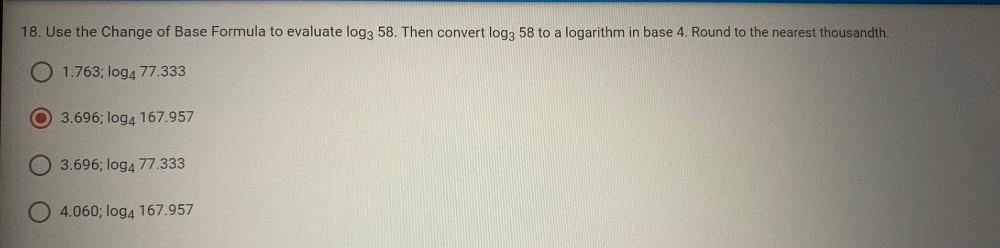Question:

# 18. Use the Change of Base Formula to evaluate log3 58. Then convert log3 58 to a logarithm in base 4. Round to the nearest thou18. Use the Change of Base Formula to evaluate log3 58. Then convert log3 58 to a logarithm in base 4. Round to the nearest thousandth. 1.763; log4 77.333 3.696; log4 167.957 3.696; log4 77.333 04.060; log4 167.957